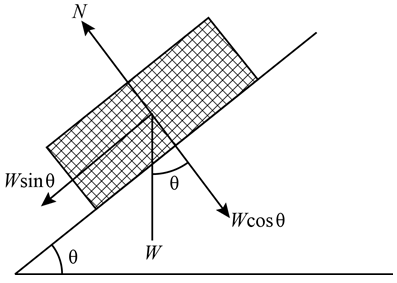# Which of the following statements is most correct?(A) The normal force is the same thing as the weight.(B) The normal force is different from the weight, but al- ways has the same magnitude,(C) The normal force is different from the weight, but the two form an action-reaction pair according to New- ton's third law.(D) The normal force is different from the weight, but the two may have the same magnitude in certain cases. θNavjyot Kalra
7 years ago

(d) The normal force is different from the weight, but the two may have the same magnitude in certain cases
Normal forces originates in the form of contact forces, in which one body exerts a force on another because of the contact between them whereas weight is the measure of the gravitational force acting on one object because of another.
Normal forces originate when the atoms of the one body exert the forces on its neighbor atoms, which may or may not be the atoms of the other body, but the forces appear due to the contact between them.
There are cases in which the magnitude of normal force is equal to the magnitude of weight such as a block resting on a horizontal table, moving horizontally on a platform. But this is not true for many cases such as the block resting on the inclined plane, because the forces originating from the contact varies from its weight.
The forces acting on the blocking resting on inclined plane is shown below:Here the magnitude of normal force is equal to the product of the cosine of the angle of inclination θ and the weight W, that is
N = W cos θ
Therefore (d) is the correct option, while the others are ruled out